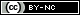## Articulo

•Citado por SciELO
•Accesos

•Similares en SciELO

## versión On-line ISSN 2007-2422

#### Resumen

LI, Xiangyue; RENDON, Luis Emilio  y  ESPINOZA, María Joselina. Considering filtration forces in the stability analysis of granular slopes. Tecnol. cienc. agua [online]. 2010, vol.1, n.3, pp.89-107. ISSN 2007-2422.

A poroelastoplastic model is used to determine collapse conditions of saturated cohesionless slopes which are subjected to a steady-state seepage field. The original coupled formulation for pore-pressure and displacement of solid skeleton is decoupled into two separate problems. The water flow of the slope is described by Laplace's equation and the stress-displacement condition is treated by the static effective-stress equilibrium equation along with an elastic-perfectly plastic constitutive relation. The seepage force field is firstly determined and the viscoplastic algorithm is then applied to calculate slope safety factors. Seepage forces in slopes are spatially variable, in contrast to the uniform seepage force distribution, assumed in the analytical solution of infinite slopes. The safety factors computed for uniform flows and infinite slopes may be close to those determined for spatially variable flow fields and two-dimensional slopes only for certain combinations of material parameters and slope geometries; under other conditions, the infinite slope solution provides significant overestimation regarding factors of safety. Non-homogeneous slopes are finally analyzed in which small variations in permeability are considered in different layers. It is demonstrated that commonly used stability analyses are far from adequate if the seepage field is taken into account only through groundwater table elevation.

Palabras llave : slope; soil; stability; seepage; water; elastoplasticity.

· resumen en Español     · texto en Español     · Español (pdf )Todo el contenido de esta revista, excepto dónde está identificado, está bajo una Licencia Creative Commons